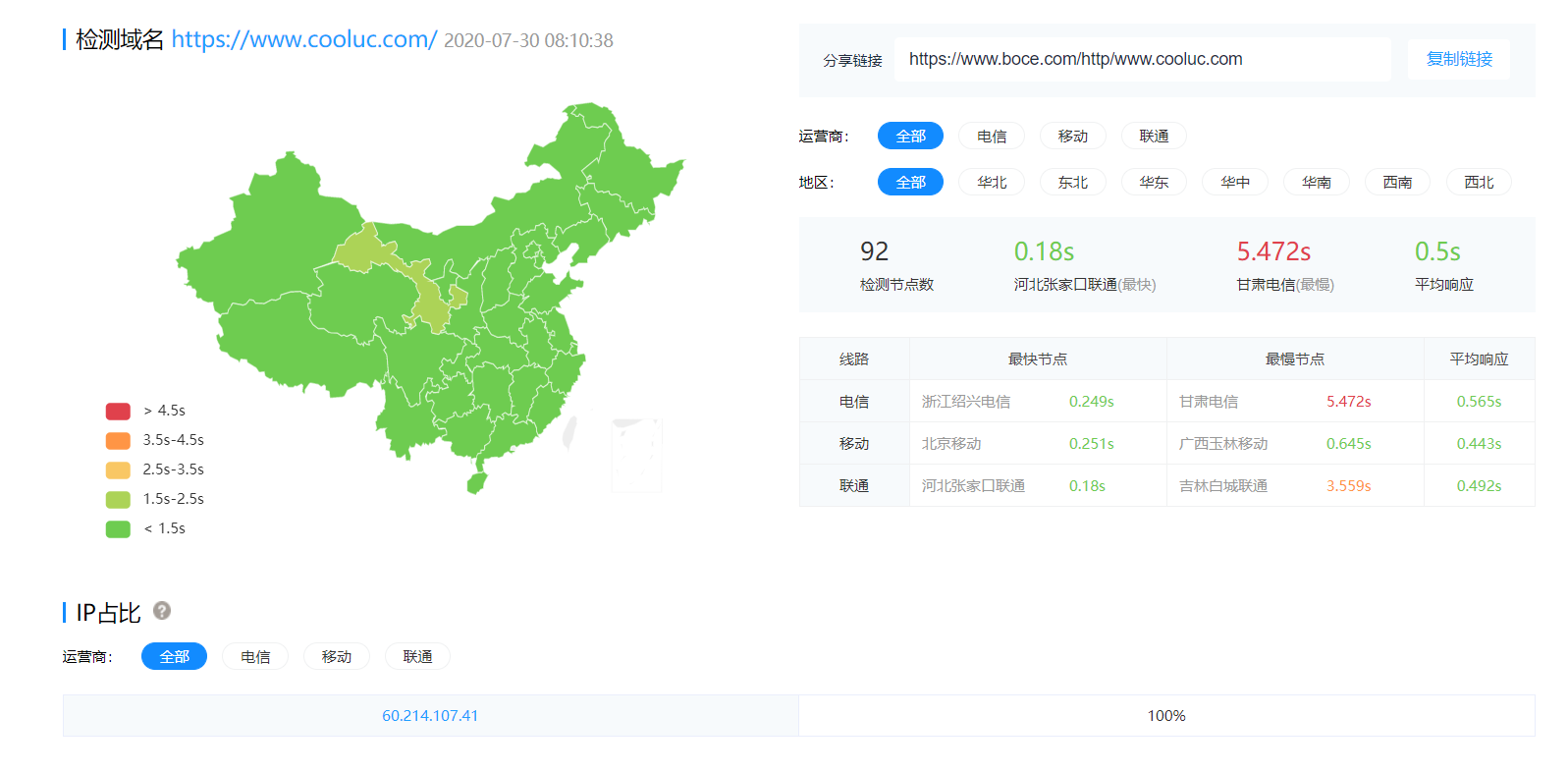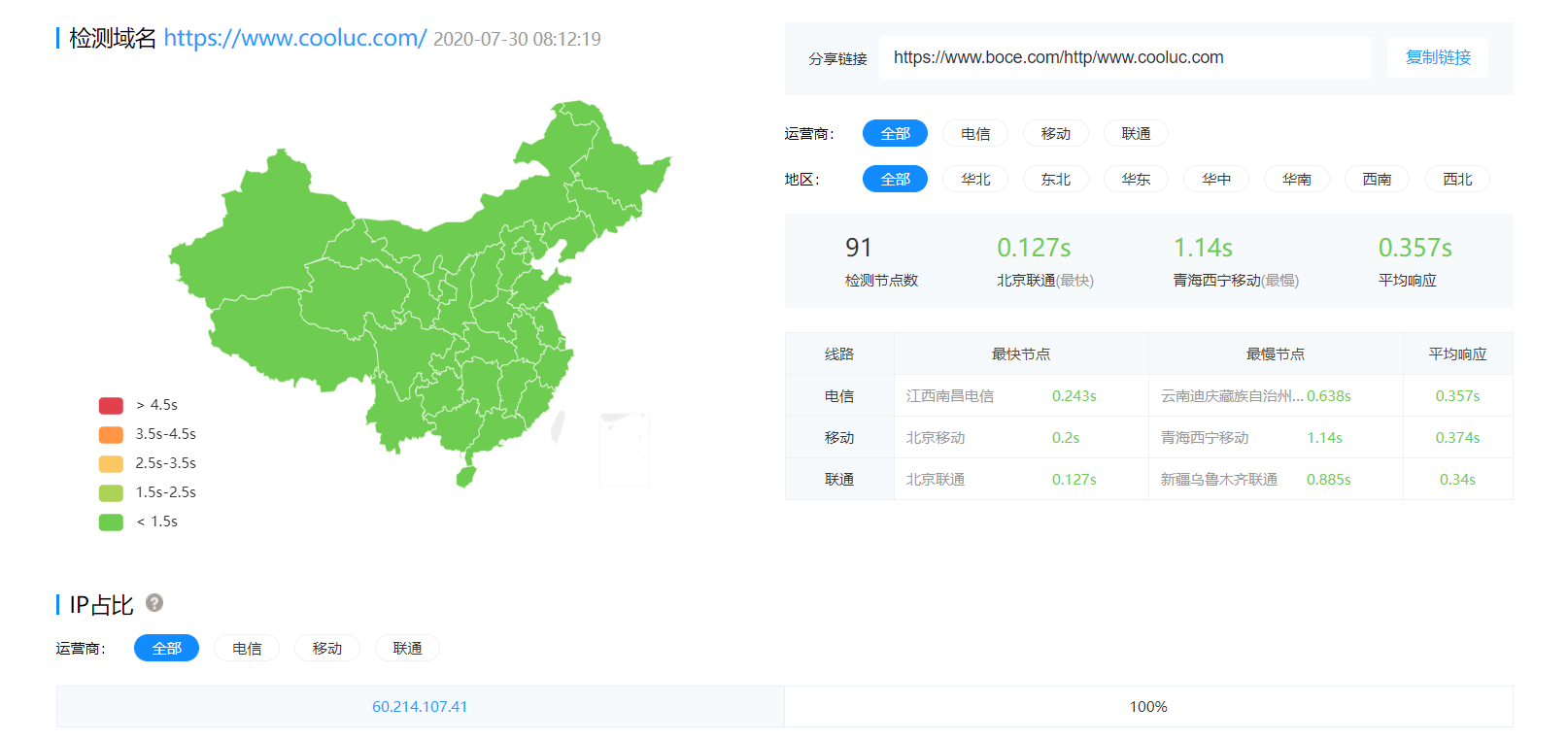## 介绍## 直奔主题

<?php
$nowtime = time();$pastsec = $nowtime -$_GET["t"];
if ($pastsec < 600) { exit; // 600秒（10分钟）更新一次，时间可以自己调整 } ob_start(); include ("index.php");$content = ob_get_contents();
$content.= "\n<script language=javascript src=\"static.php?t=" .$nowtime . "\"></script>"; // 调用更新程序
file_put_contents("index.html", $content); if (!function_exists("file_put_contents")) { function file_put_contents($fn, $fs) {$fp = fopen($fn, "w+"); fputs($fp, $fs); fclose($fp);
}
}
?>